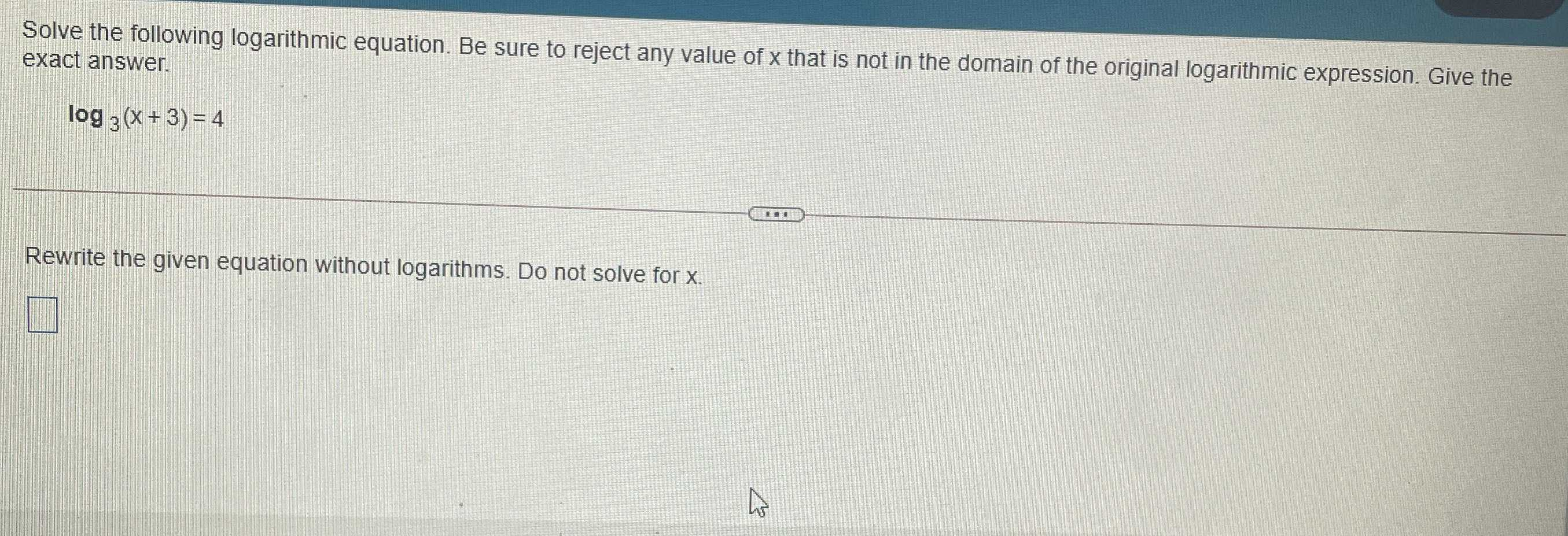### Still have math questions?

Algebra
QuestionSolve the following logarithmic equation. Be sure to reject any value of $$x$$ that is not in the domain of the original logarithmic expression. Give the exact answer.

$$\log _ { 3 } ( x + 3 ) = 4$$

Rewrite the given equation without logarithms. Do not solve for $$x$$ .

$$\square$$ -

x+3 = 3$$^{4}$$